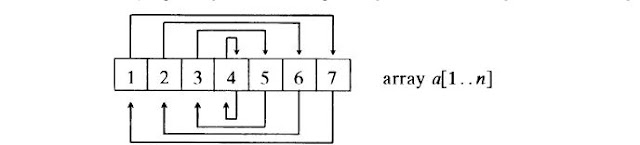### How to Reverse an Array in Java With and Without Using Additional Array?

In this article, I will explain how to solve the given problem. We are going to solve this problem in two ways and also we will discuss which approach is efficient.

Problem:
Reverse the Given Array.

Solution:
You can solve this problem in two ways.
1. Using an additional array which has same size as give array and type.
2. Without using the additional array.

Let's see the first approach.

Consider we have given an integer array with 10 elements i.e {1,2,3,4,5,6,7,8,9,10}. In this approach, we take an empty integer array which is of same size i.e 10, and we traverse the given array from tail to begin ( from last index to begin index) and insert the element in the additional array from begin to tail.

Hence the 10th element from original array goes into 1st position of additional array, 9th element goes into 2nd position, 8th element goes to 3rd position and so on.

Solution Step by Step:

1. Given original array
`int[] a = {1,2,3,4,5,6,7,8,9,10};`
`int[] b = new int[a.length]; // same type as original array (int), same size (10)`
3. traverse the original array from back and add the elements into additional array from start.
```int j = 0;

for(int i = a.length-1; i >= 0; i--) {

b[j++] = a[i];

}```
4. print the array, use Arrays.toString(b) for this purpose.
`System.out.println(Arrays.toString(b));`
The complete program :
```package com.speakingcs.arrays;

import java.util.Arrays;

public class ArrayReverse {

public static void main(String[] args) {

ArrayReverse ar = new ArrayReverse();

int[] a = { 1, 2, 3, 4, 5, 6, 7, 8, 9, 10 };
ar.reverse(a);

}

private void reverse(int[] a) {

int[] b = new int[a.length];
int j = 0;

// array index starts from 0,
// so i should be initialized to array length - 1.
for (int i = a.length - 1; i >= 0; i--) {
b[j++] = a[i];
}

System.out.println(Arrays.toString(b));

}

}
```

The problem with this approach is, every time we need to create an additional array of same size and same type. Imagine what if we have an array of size more than million, so our problem creates a temporary array of same size which wastes so much of memory.

space complexity is O(n) - for additional array size n.
time complexity is also O(n). - time take to traverse the array depends on number of elements in the array(n).

Let's see the 2nd approach

In this approach, we are not going to use any additional array, instead we swap first and last elements of the array, 2nd and last but one element and so on.For this purpose, we are going to take two counters, one is pointing to start of the array ( i = 0), another is pointing to end of the array ( j = n-1). So while traversing the array from begin to end, we swap the elements at indexes i and j and also the counter i moves forward, where as counter j moves backwards.

Now we got to know, how to solve the problem, but we don't know when to stop the array iteration. We need to stop the array iteration, once we reach the middle of the array.

Solution Step by Step:

1. Given the original array
`int[] a = {1,2,3,4,5,6,7,8,9,10};`
2. Find the middle of the array
` int middle = a.length / 2;`
3. Take two counters i, j and initialize
`int i = 0, j = a.length - 1;`
4. Traverse the array from begin to middle and swap the elements at the indexes i , j.
```int temp = 0;
// counter i goes forward, counter j moves backwards.

for(; i < middle; i++,j--) {
//swapping the elements.
temp = a[i];

a[i] = a[j];

a[j] = temp;

}```
5. Finally print the array.
`System.out.println(Arrays.toString(a));`

The complete program is:
```package com.speakingcs.arrays;

import java.util.Arrays;

public class ArrayReverse {

public static void main(String[] args) {

ArrayReverse ar = new ArrayReverse();

int[] a = { 1, 2, 3, 4, 5, 6, 7, 8, 9, 10, 11};
ar.reverseArray(a);

}

private void reverseArray(int[] a) {
int middle = a.length /2;
int i = 0, j = a.length - 1, temp = 0;

for(; i < middle; i++,j--) {
temp = a[i];
a[i] = a[j];
a[j] = temp;
}

System.out.println(Arrays.toString(a));
}
}
```

In this approach, we are not using any additional array. For swapping purpose, we are using one temporary variable. The no of iterations are also n/2 i.e half of the array length.

space complexity is O(n) - for temporary variable.
time complexity is also O(n/2) = O(n). - time take to traverse the array depends on number of elements in the array(n).